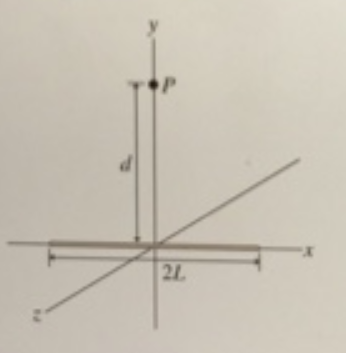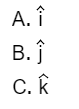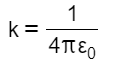# Problem: A charged wire of negligible thickness has length 2L units and has a linear charge density λ. Consider the electric field E→ at the point, P, a distance of above the midpoint of the wire. Part A. The field  E points along one of the primary axes. Which one? Part B. What is the magnitude E of the electric field at point P? Throughout this part express your answers in terms of the constant, k, defined by Express your answer in terms of L, λ, d, and k.

###### FREE Expert Solution

Part A

The cosine components in the x-direction will cancel each other.

The y-components are in the same direction.###### Problem Details

A charged wire of negligible thickness has length 2L units and has a linear charge density λ. Consider the electric field $\stackrel{\to }{\mathrm{E}}$ at the point, P, a distance of above the midpoint of the wire.Part A. The field  E points along one of the primary axes. Which one?Part B. What is the magnitude E of the electric field at point P? Throughout this part express your answers in terms of the constant, k, defined by